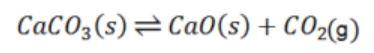• Call Now

1800-102-2727•

# Heterogeneous Equilibrium

## Overview

A heterogeneous equilibrium occurs when a system's state of equilibrium contains components from various phases. For example, if we put water and ice in a container at a temperature that allows both phases to exist at the same time, both water and ice are present in an equilibrium state. Heterogeneous equilibrium is the name given to this situation.

• The reacting species mix with one another to generate products in a chemical reaction.
• When such a reaction takes place in a closed container, we frequently discover that it is not finished. The reaction mixture contains both the product and reactant species after a period of time.
• Furthermore, if both the pressure and the temperature remain constant, the concentration of these species becomes constant over time.
• The reaction is considered to be in a state of equilibrium when the conditions are met.
• The products and reactants are in distinct phases in a heterogeneous system comprising a reversible state of reaction.

Here is an example of a reversible reaction using CaCO3 decomposition. Decomposition of CaCO3 yields CO2 and CaO . When the rate at which CaCO3decomposes is the same as the rate at which the product decomposes, the reactant is formed. At this point, a state of equilibrium is achieved.## Examples

Steam reacts with red hot carbon to yield hydrogen gas and carbon monoxide gas.

H2O (g) + C (s) ⇌ H2(g) + CO(g)

Carbon monoxide gas is produced when carbon dioxide gas reacts with solid carbon at equilibrium.

CO2 (g) + C (s) ⇌ 2 CO(g)

Solid ferrous oxide reacts with carbon monoxide gas to form solid iron and carbon dioxide gas. This is an example of a heterogeneous equilibrium.

FeO (s) + CO (g) ⇌ Fe (s) + CO2(g)

• The equilibrium constant for heterogeneous reactions is written differently as compared to the equilibrium constant for homogeneous processes.
• Take the heat disintegration of CaCO3 into CaO and CO2, for illustration. The equilibrium constant for the disintegration of the reactant into its products is fully reliable on the gaseous component, as the liquid and solid reactants are assumed to be constant.Because the components CaCO3 and CaO are solids, their molar concentration does not change during the reaction. As a result, the equilibrium constant can be expressed as follows:In terms of Kp, we can also write

The partial pressure is denoted by the letter p. In other words, we can say that in the equilibrium reaction between CaO and CaCO3, there is a constant partial pressure of CO2at a certain temperature.

Similarly: for equation CO2(g) + C (s) ⇌ 2 CO(g)

Equilibrium constant Kc = [ CO]2 / [CO2]

Physical Change Equilibrium: Phase changes are also heterogeneous equilibrium systems. We know that the states of matter are solid, liquid, and gas, hence several types of equilibrium are possible between

Solid – Gas Equilibrium

Liquid – Gas Equilibrium

Solid – Liquid Equilibrium

Solid-Gas Equilibrium: This state of equilibrium occurs when chemicals such as ammonium chloride, camphor,  iodine, and others sublimate when heated or even at room temperature. They go straight from liquid to vapour or gaseous without going through the liquid state.I2

(s) ⇋ I2 (g)

Camphor (s) ⇋ Camphor (g)

NH4Cl (s) ⇋ NH4Cl (g)

Liquid - Gas equilibrium: The evaporation of water in a closed container at room temperature can be used to illustrate the liquid-gas equilibrium.
H2O (l) ⇋ H2O (g)

Solid – Liquid Equilibrium: The equilibrium between ice and water in a thermo flask at 0°C and 1 atm pressure. In this situation, the melting of ice and the freezing of water occur at the same time. This can be stated as follows in terms of its equation:
H2O (s) H2O (l)Talk to our expert
Resend OTP Timer =
By submitting up, I agree to receive all the Whatsapp communication on my registered number and Aakash terms and conditions and privacy policy